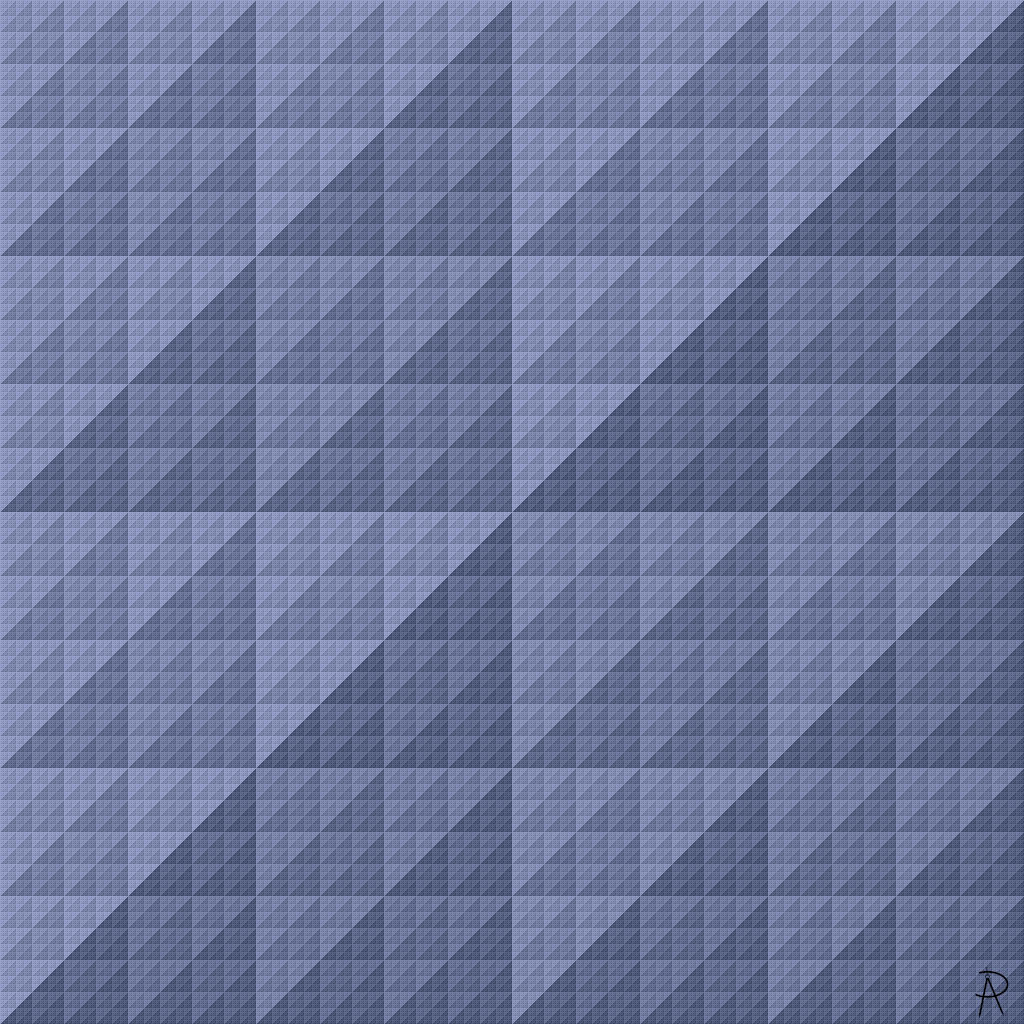Carry on My Wayward Sum Digital Print 2021

This image is a visualization of the number of carries needed when adding two integers using their binary representations. Carry counts for the sums of the integers between 0 and 1023 are shown. The addition of 0 and 0 is in the top left and requires zero carries, represented by a light color. The addition of 1023 and 1023 is shown at the lower right and requires 10 carries, represented by a dark color.

### Background and Inspiration

I came across a programming exercise that asked one to write an algorithm to count the number of carries needed to add two numbers expressed in binary. After coding an algorithm, I decided to see if there was any interesting structure in the carry counts. The above image is the surprising result.

#### Number Representations

Integers can be represented in many ways. For example, the number 5 can be represented in English as five, the digit 5, as hand showing five fingers, a V in Roman numerals, or tally marks. Five can also be represented in binary as 101.

#### Decimal Representation

We use a base ten (decimal or denary) system when representing numbers, which uses the ten symbols 0, 1, 2, 3, 4, 5, 6, 7, 8, and 9. The value and position of a digit in the representation determines its contribution to the value of a number. The value of a digit is multiplied by the value of its position. The value of the positions start at one in the right-most position and increase by powers of ten as we move from right to left.

For example, the value represented by the number 456 is given by \begin{align} 456 &= 400 + 50 + 6\cr &= 4 \times 100 + 5 \times 10 + 6 \times 1\cr &= 4 \times 10^2 + 5 \times 10^1 + 6 \times 10^0.\cr \end{align} Because $$10^2 = 100$$ we say 4 is in the one-hundreds position. Similarly, we say 5 is in the tens position and 6 is in the ones position.

#### Positional Representation

While we commonly represent numbers using a positional number system in base ten, the base is arbitrary. In a positional system, the value a digit contributes to the total value of a number depends on the position of that digit. The values of the positions are the powers of the base. Given a specific base $$b$$, the only valid digits in that base are $$0$$, $$1$$, $$\ldots$$, and $$b-1$$, each of these digits are represented by a unique symbol. In situations needing clarification we can write the base as a subscript following the number to specify its base such as $$456_{\text{(ten)}}$$. In addition to base ten, other common bases include two (binary), eight (octal), and sixteen (hexadecimal).

Not all representation systems for numbers are positional. For example, Roman numerals do not use a positional system, which makes arithmetic more complicated. For example, fifty-five is expressed as 55 in base ten and LV in Roman numerals because 55 = 50 + 5 = L + V = LV. Similarly, 89 = 50 + 10 + 10 + 10 + 9 = 50 + 10 + 10 + 10 + (10-1) = L + X + X + X + I + X. Adding 55 and 89 results in 144. The equivalent in Roman numerals this would be LV + LXXXIX = CXLIV. In a positional system, a larger number of digits indicates a number with a larger value. That is not true in general for the Roman numeral system.

#### Binary Representations

In binary representations of numbers, we only use two symbols, 0 and 1, called bits (a shortening of binary digit). Because it is easier to distinguish between two possible states, binary is used in computer systems to represent data such as numbers. As in base ten, we use the positions of a binary number to determine the value a bit contributes to a number's value. For example, the value of the binary number 111001000 is determined by $1 \times 2^8 + 1 \times 2^7 + 1 \times 2^6 + 0 \times 2^5 + 0 \times 2^4 + 1 \times 2^3 + 0 \times 2^2 + 0 \times 2^1 + 0 \times 2^0.$ Note that we are either including or excluding adding specific powers of two depending on the bit in a given position. When the bit in a position is a 1, we add the corresponding power of two, otherwise we add nothing. Thus, \begin{align} 111001000_{\text{(two)}} &= 1 \times 2^8 + 1 \times 2^7 + 1 \times 2^6 + 0 \times 2^5 + 0 \times 2^4 + 1 \times 2^3 + 0 \times 2^2 + 0 \times 2^1 + 0 \times 2^0 \cr &= 2^8 + 2^7 + 2^6 + 2^3 \cr &= 256 + 128 + 64 + 8 \cr &= 456_{\text{(ten)}} \cr &= 400 + 50 + 6 \cr &= 4 \times 100 + 5 \times 10 + 6 \times 1\cr &= 4 \times 10^2 + 5 \times 10^1 + 6 \times 10^0. \cr \end{align}

#### Adding Numbers in Base Ten

If we add two numbers in base 10, such as 55 and 89, we add from left to right, one position at a time. In some cases, the sum of the two digits exceeds the base 10, which results in a carry. The carry indicates we have an additional power of 10 to add in the next position. When we add 5 and 9, we get 14 = 10 + 4. Thus we write a 4 in the sum and add an additional 10, resulting in a carry. Similarly, we get 4 with a carry of 1 when adding 1+5+8.

Position 102 101 100
Position 100 10 1
Carry 1 1
5 5
+ 8 9
Result 1 4 4

#### Adding Numbers in Base Two

The process of adding numbers base two is similar to base ten, except that we only use the two symbols 0 and 1. As in the base ten example, we sometimes need to add three bits when adding two binary numbers. The third bit is a possible carry from the addition of the previous bits. The total number of ones added is either zero (0), one (1), two (10), or three (11). These possibilities are shown in the table below.

 0 0 0 1 0 1 1 1 1 1 1 1 0 0 1 1 0 0 1 1 + 0 + 1 + 0 + 1 + 0 + 1 + 0 + 1 0 1 1 1 0 1 1 0 1 0 1 1

For example, when adding 1 and 1 with an input carry of 0, the addition generates a carry, resulting in a final value of 10, as shown above in column 4.

Now consider adding two binary numbers. In each position left of the ones position there is a possible carry from the previous calculation. For example, to add fifty-five and eighty-nine we first need to represent each as binary. \begin{align} 55_{(\text{ten})} &= 32 + 16 + 4 + 2 + 1 \cr &= 2^5 + 2^4 + 2^2 + 2^1 + 2^0 \cr &= 1 \times 2^5 + 1 \times 2^4 + 0 \times 2^3 + 1 \times 2^2 + 1 \times 2^1 + 1 \times 2^0 \cr &= 110111_{(\text{two})} \end{align} and \begin{align} 89_{(\text{ten})} &= 64 + 16 + 8 + 1 \cr &= 2^6 + 2^4 + 2^3 + 2^0 \cr &= 1 \times 2^6 + 0 \times 2^5 + 1 \times 2^4 + 1 \times 2^3 + 0 \times 2^2 + 0 \times 2^1 + 1 \times 2^0 \cr &= 1011001_{(\text{two})}. \end{align}

Adding 55 and 89 in binary results in 7 carries as shown below.

 1 1 1 1 1 1 1 Carry 1 1 0 1 1 1 55 + 1 0 1 1 0 0 1 89 1 0 0 1 0 0 0 0 144

Not all binary additions result in carries. For example, adding 42 and 85 results in no carries as shown below.

 Carry 1 0 1 0 1 0 42 + 1 0 1 0 1 0 1 85 1 1 1 1 1 1 1 127

Other binary additions result in only a few carries. For example, adding 42 and 42 results in three carries as shown below.

 1 1 1 Carry 1 0 1 0 1 0 42 + 1 0 1 0 1 0 42 1 0 1 0 1 0 0 84

This artwork contains the number of these carries when adding pairs of integers between 0 and 1023 inclusive. Note that 1023 is the largest number one can represent using 10 bits and has a binary representation 1111111111.

One question I had when working on this was what did the patterns look like for specific carry counts. The series of images for carry counts from 0 to 10 are shown in the series of figures below. The resulting patterns are related to the Sierpinski triangle.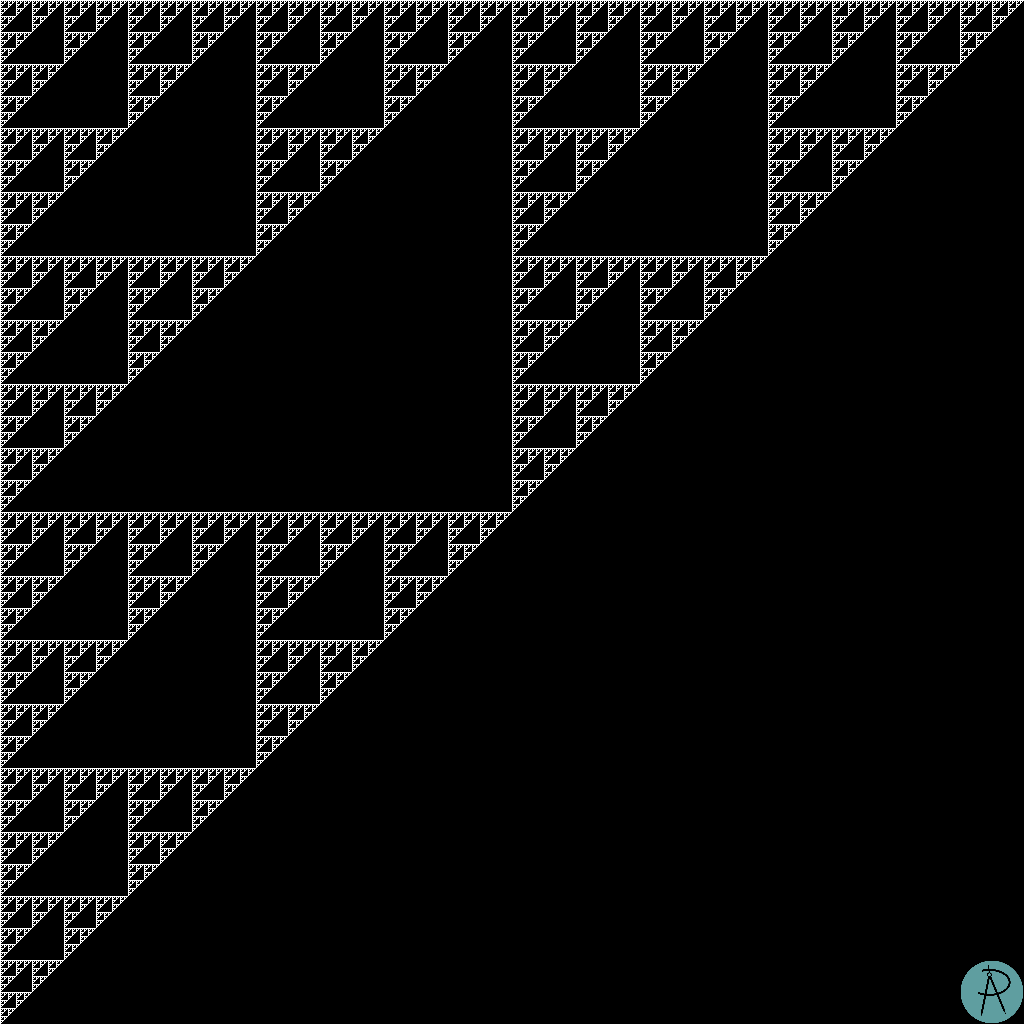The sums where the carry count is 0. The total number of such sums is 59049 (about 5.6%).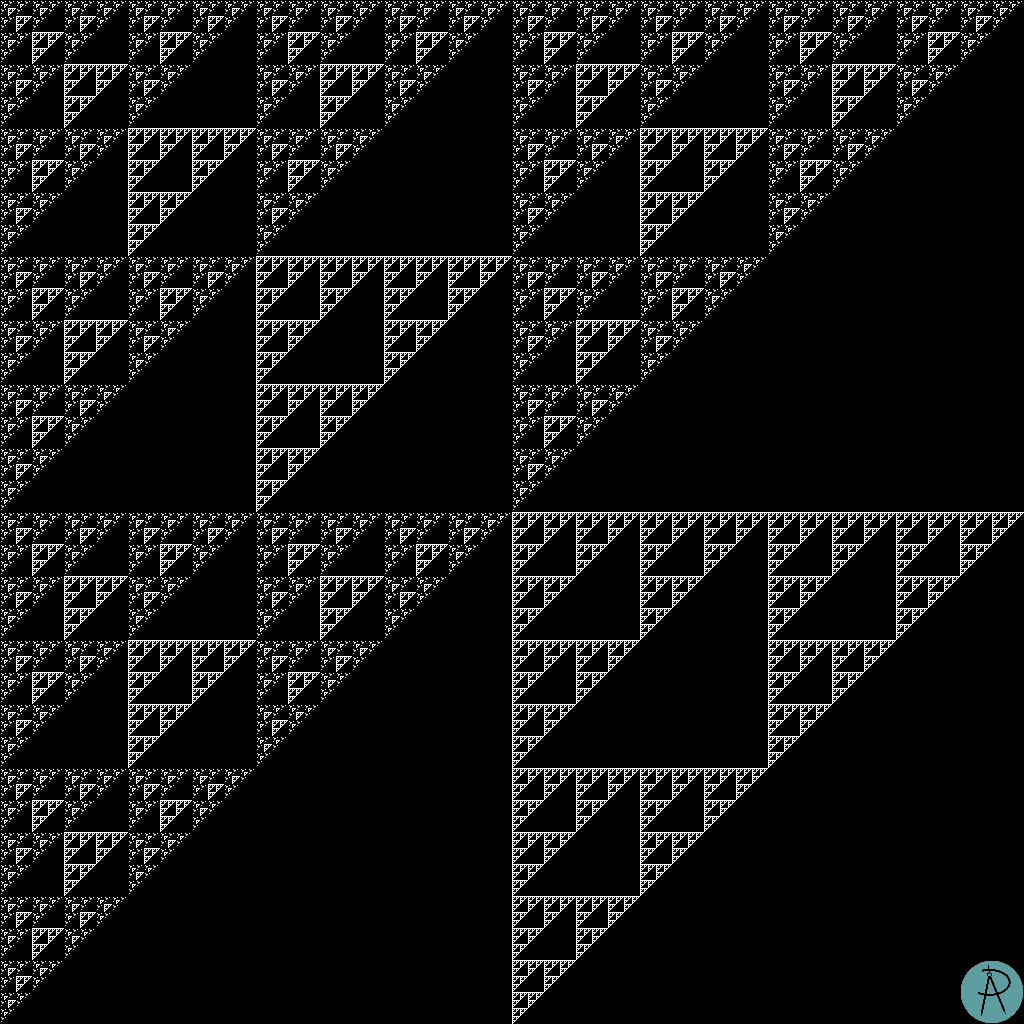The sums where the carry count is 1. The total number of such sums is 78732 (about 7.5%).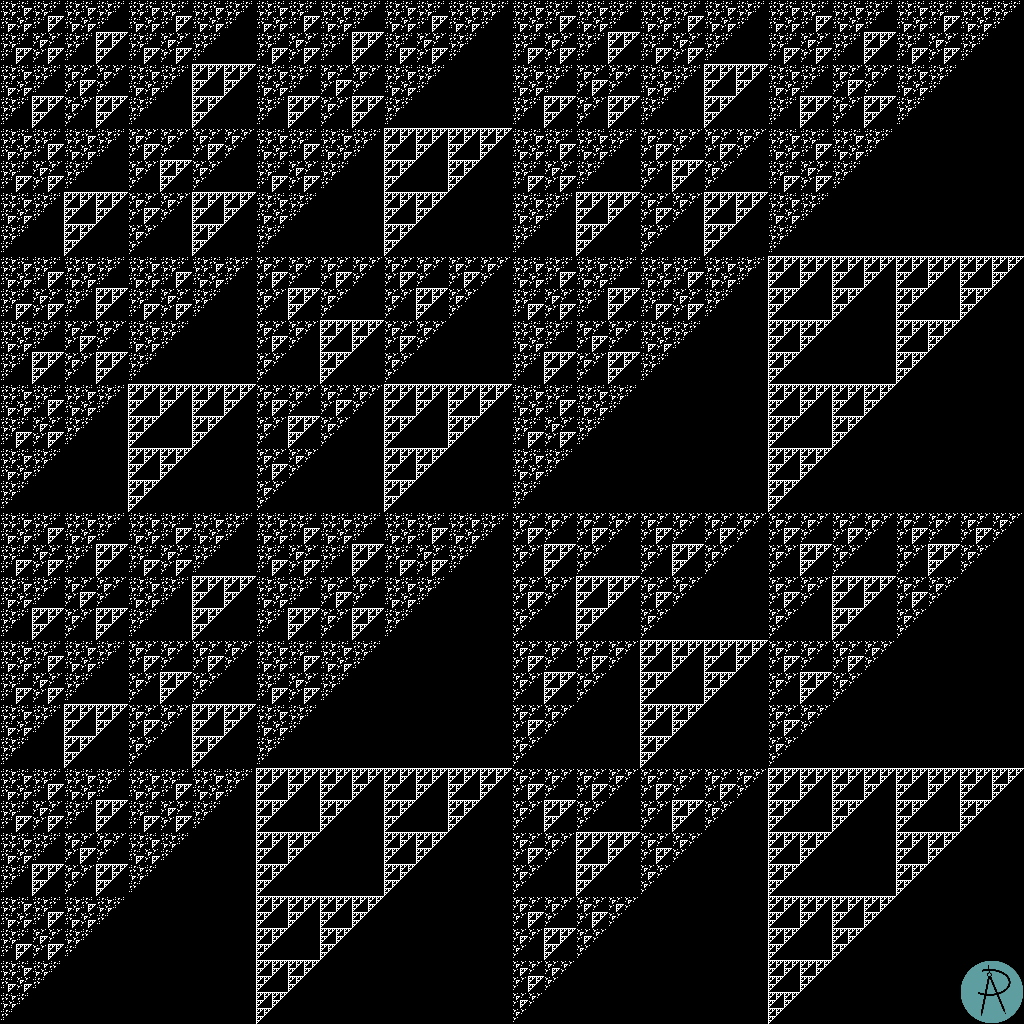The sums where the carry count is 2. The total number of such sums is 110079 (about 10.5%).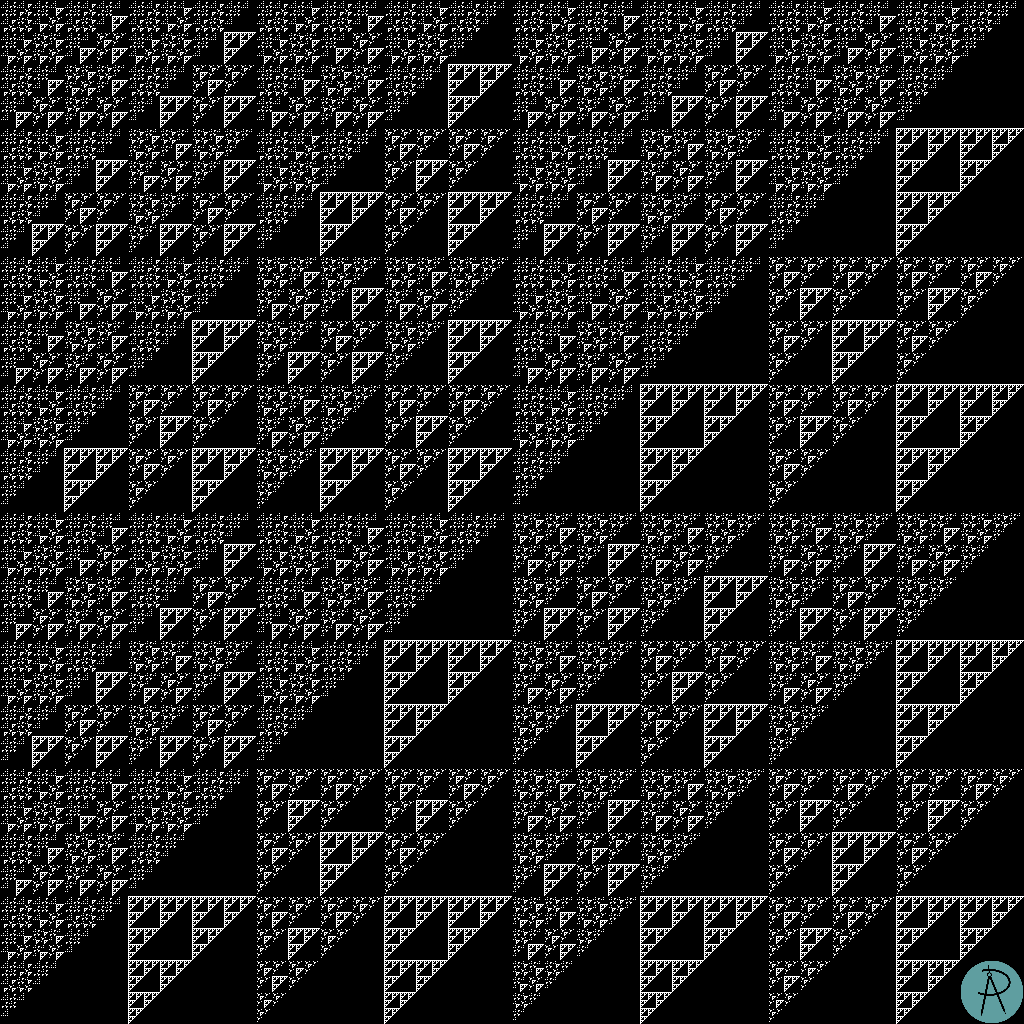The sums where the carry count is 3. The total number of such sums is 134784 (about 12.9%).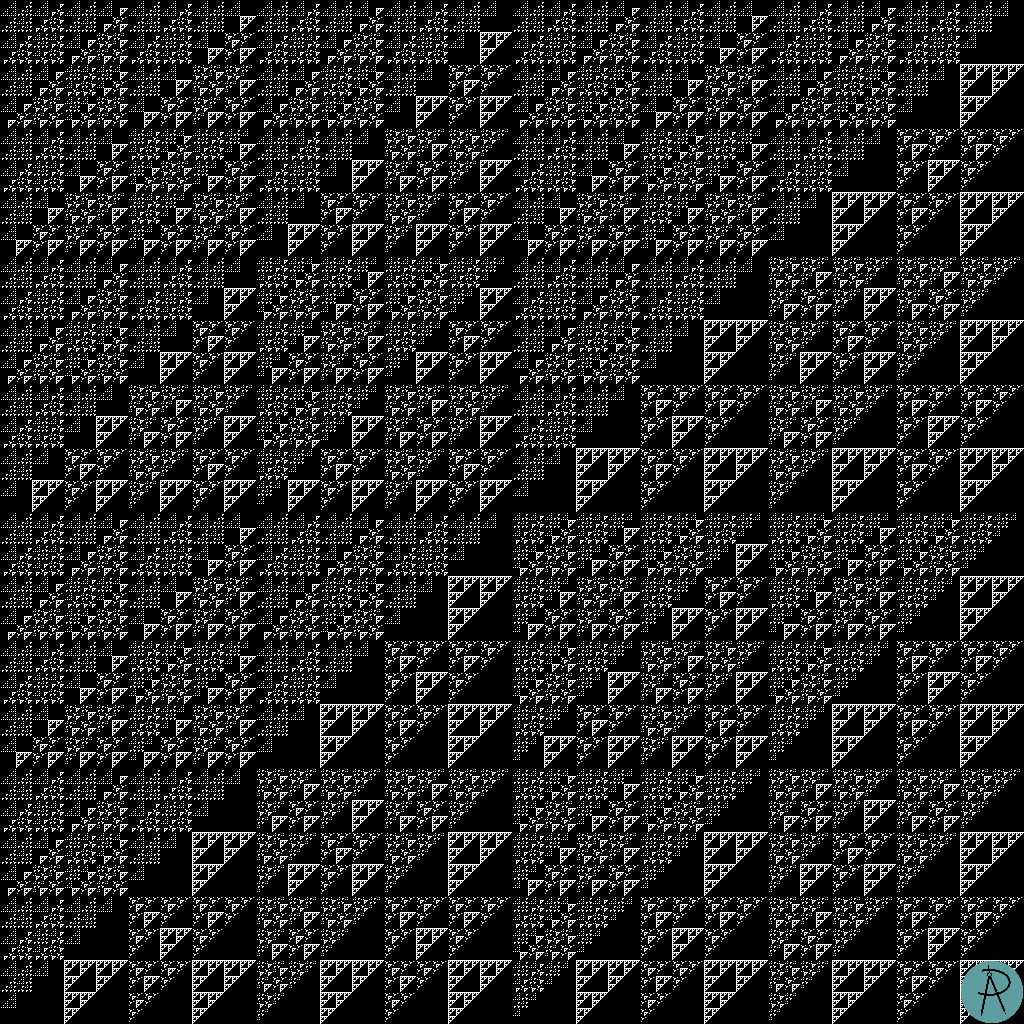The sums where the carry count is 4. The total number of such sums is 147690 (about 14.1%).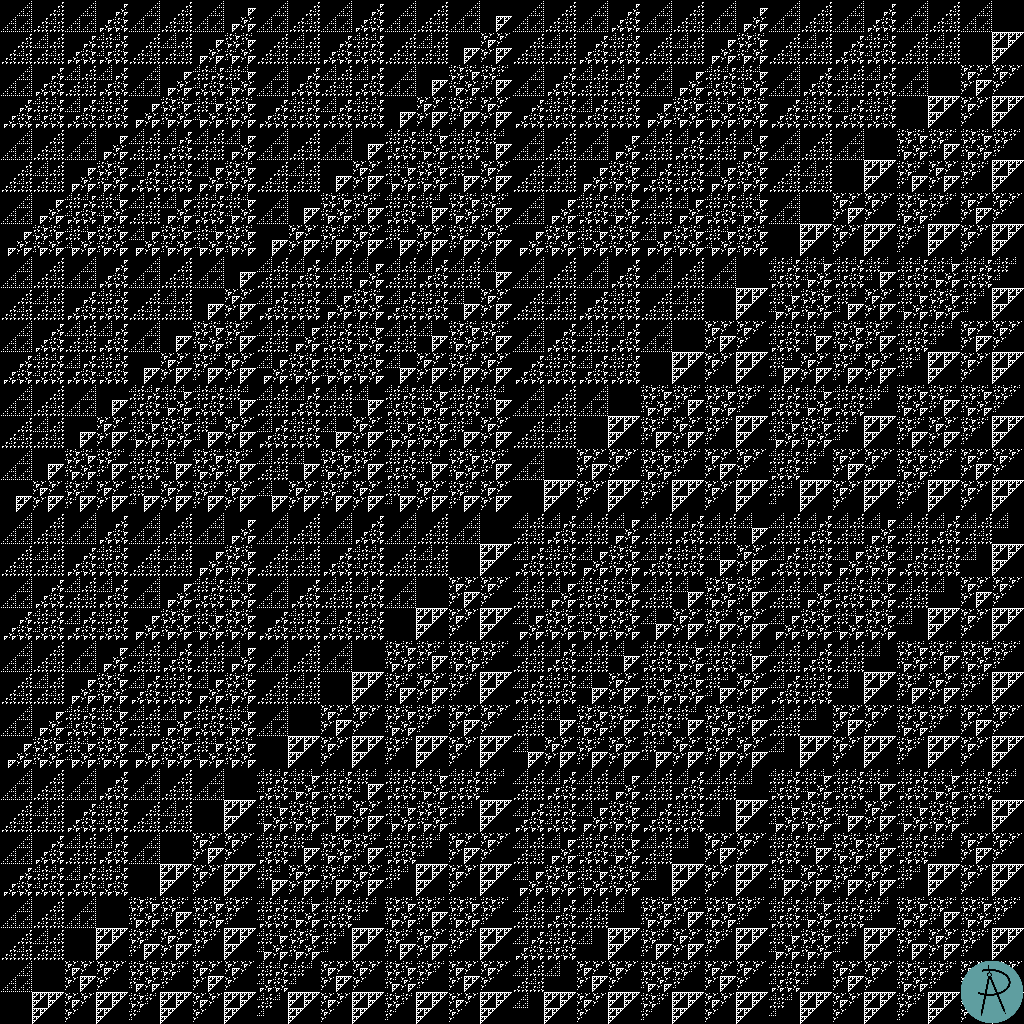The sums where the carry count is 5. The total number of such sums is 146104 (about 13.9%).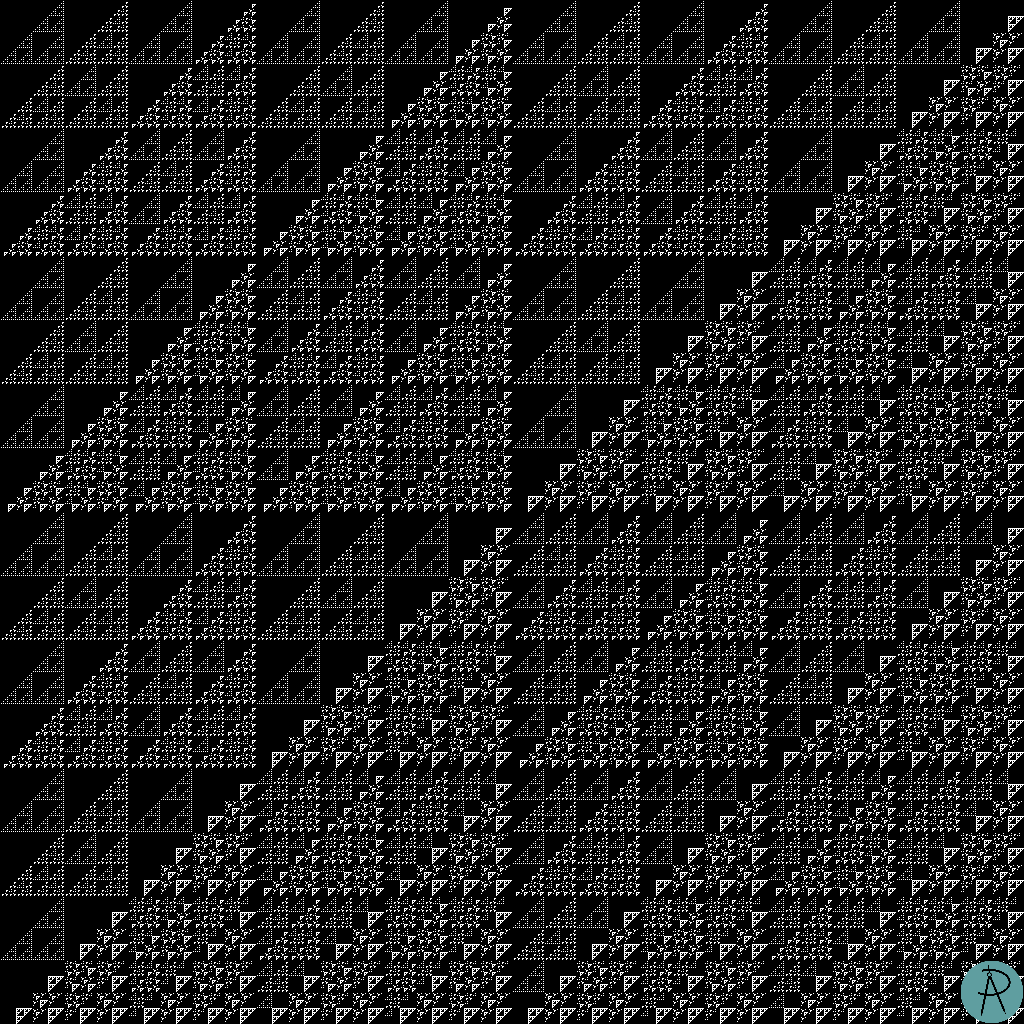The sums where the carry count is 6. The total number of such sums is 130542 (about 12.4%).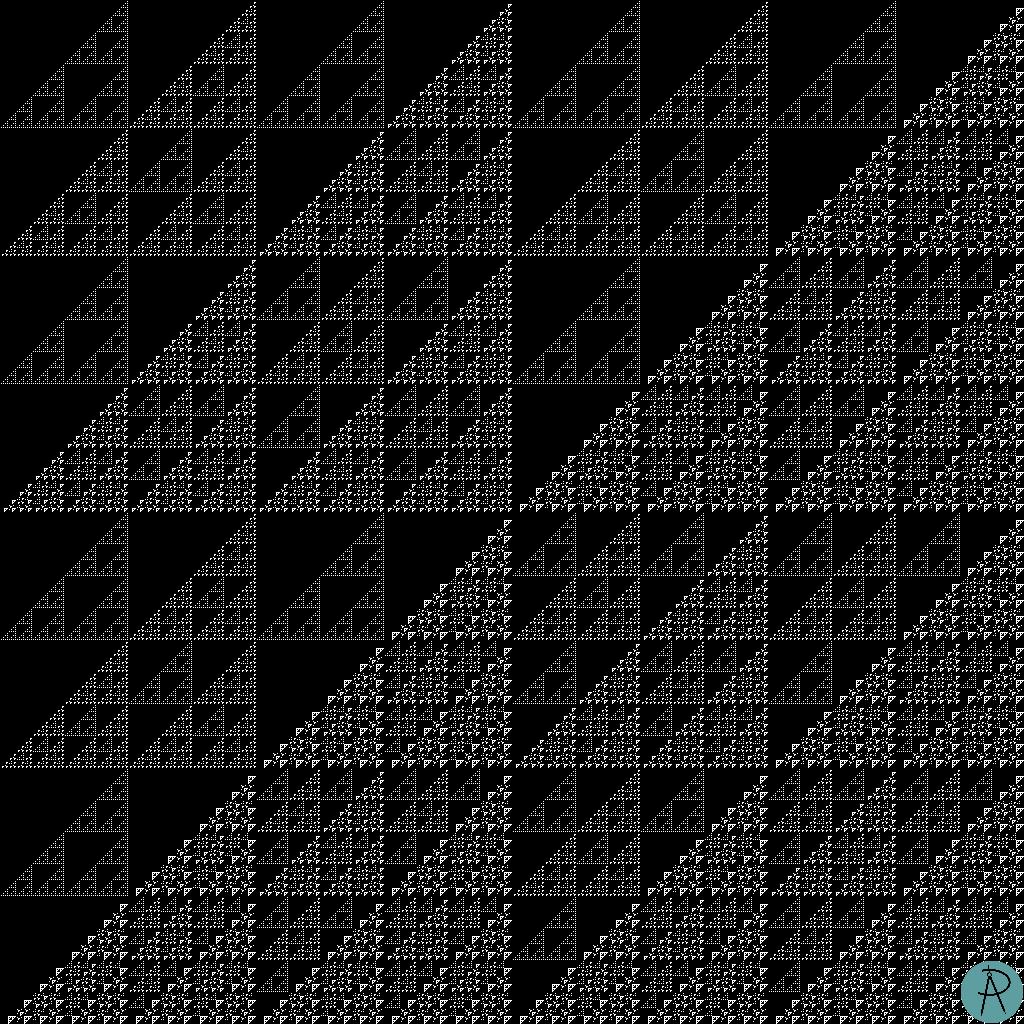The sums where the carry count is 7. The total number of such sums is 104544 (about 10.0%).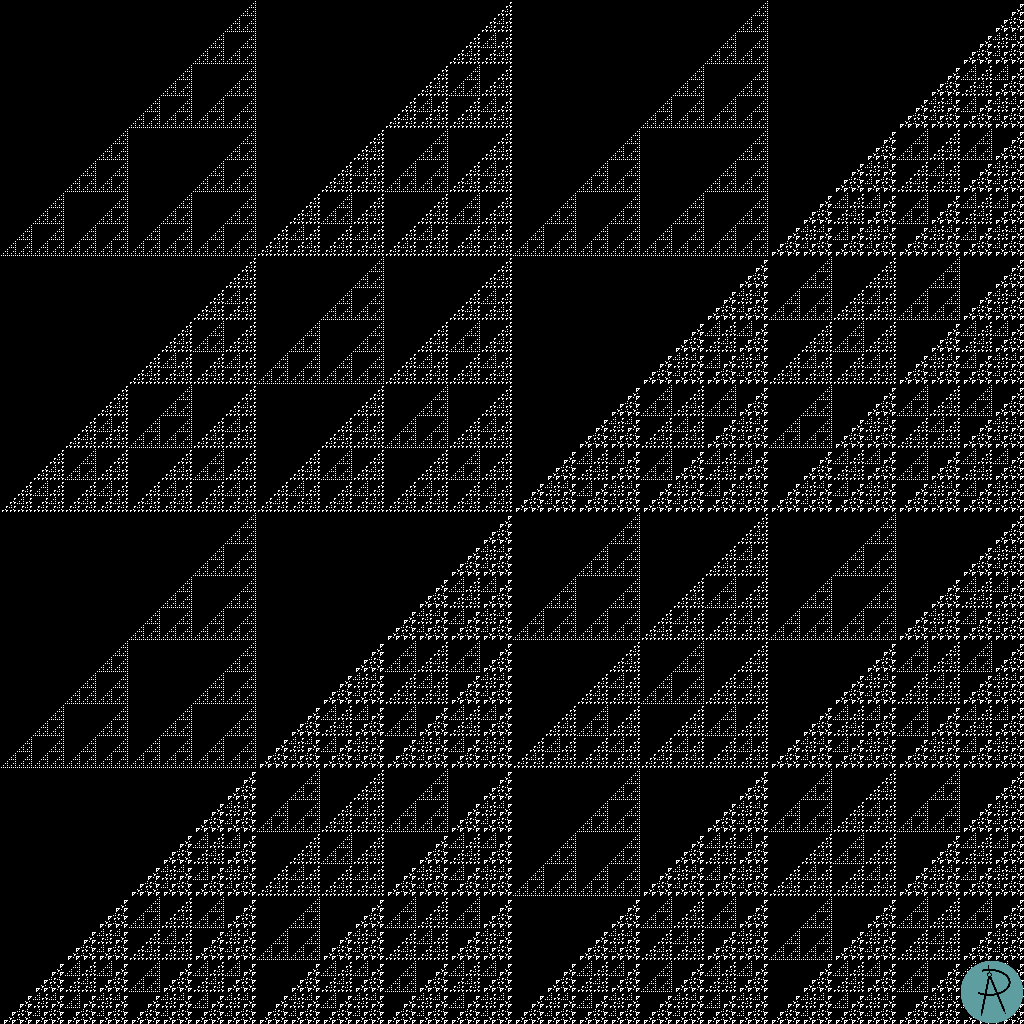The sums where the carry count is 8. The total number of such sums is 73629 (about 7.0%).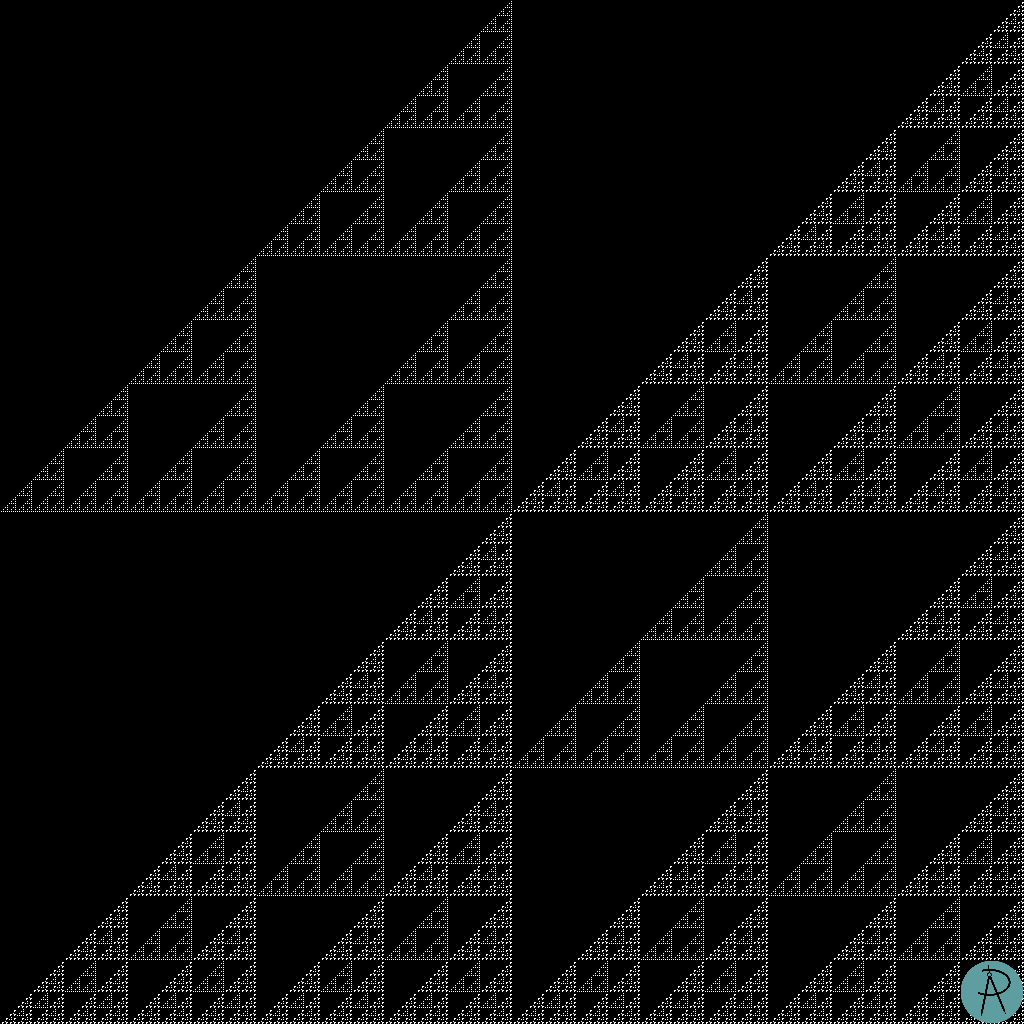The sums where the carry count is 9. The total number of such sums is 43740 (about 4.2%).The sums where the carry count is 10. The total number of such sums is 19683 (about 1.9%).